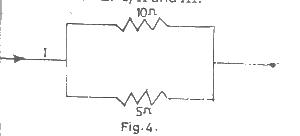# In the Fig above, Current I passes through the combination, if the power dissipated in the 5 ohm resistor is 40W, then the power dissipated in the 10 ohm re...

Question 1In the Fig above, Current I passes through the combination, if the power dissipated in the 5 ohm resistor is 40W, then the power dissipated in the 10 ohm resistor is